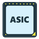Short

# BTCCNY - Zoom out: Bottom is Near (in time)

BTCCHINA:BTCCNY   Bitcoin / Chinese Yuan
5998 views
5998
After the near term bull trap finishes, price should continue down to bottom to complete Wave C and start new cycle to ATH , with bottom target of 1390.

Target of 1390 calculated by determining estimated end points of Waves C, V, 5 and v (and later little 5), by looking for fibonacci clusters that coincide with all waves (and little wave 5 once little waves 3 and 4 are complete):
(i) Wave C (of largest degree): Wave C = Waves A-B x 0.50 (50%) = 1390
(ii) Wave V (of Wave C of largest degree): Wave V = Waves I - III x 0.386 (38.6%) = 1390
( iii ) Wave 5 (of Wave V of Wave C of largest degree): Wave 5 = Wave 1-3 x 1.618 (161.8%) = 1390
(iv) Wave v (of Wave 5 of Wave V of Wave C of largest degree): Wave v = Waves i-iii x 1.618 (161.8%) = 1390
(v)And determined estimated end point of wave V of largest degree Wave C and found that it has a fibonacci end point that coincides with the other named waves, at 1390.

Specifically, if Wave V = 38.2% of Wave I-III, then estimated end point of 1390.